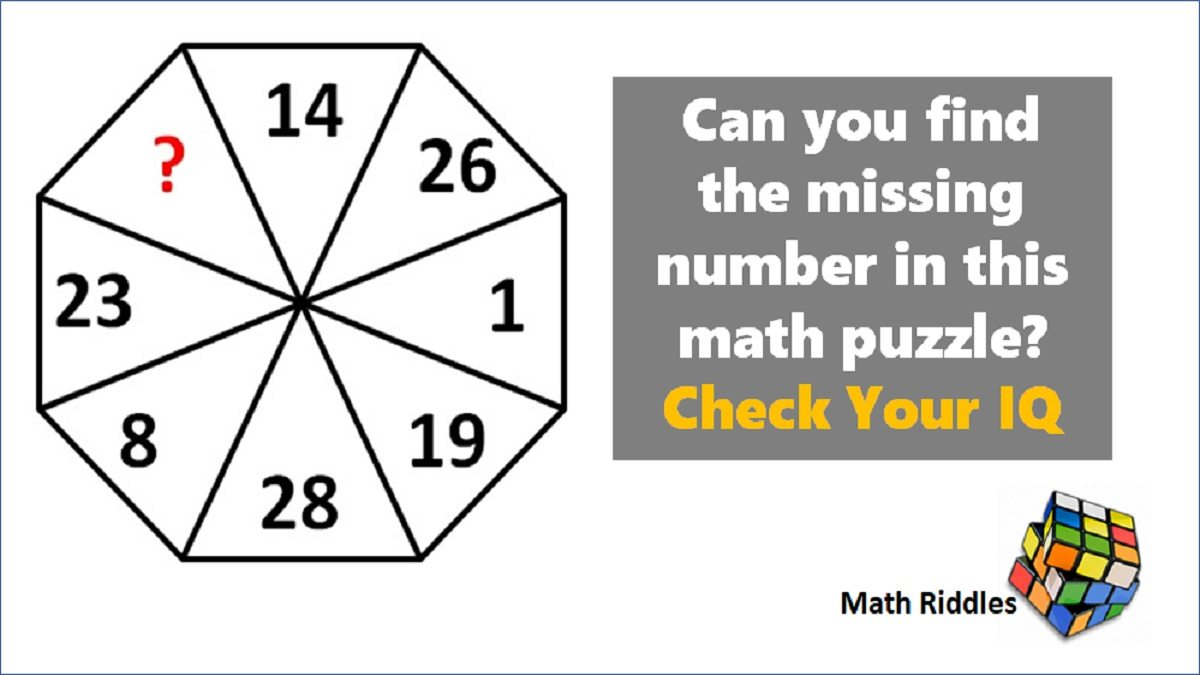# Math Riddles: Can you find the missing number in this math puzzle? Check Your IQ

Math Riddles Challenge: Can you find the missing number in this math puzzle? Check Your IQMath Riddles: Can you find the missing number in this math puzzle? Check Your IQ

Math Riddles are fun and challenging. One who enjoys math will love to solve this math problem. Here, we have a very hard to solve missing number puzzle that will help you gauge your IQ level and math aptitude. Let us see if you can figure out the number pattern in this puzzle and find the missing number in 30 seconds.

## Solution

Logic Puzzle #1

Let us figure out the number pattern and placement.

So, we have established the series here. Similarly, we shall apply the logic and add +1 to 28 and get the missing number.

## Tell us in comments: Did you solve this find missing number puzzle correctly in 30 seconds?

Check out more math puzzles!

Also Read: Math Riddles: Can You Find the Logic in this Magic Square 3×3?

Also Read: Math Riddles: Can you solve these 3x3 Magic Squares in 30 seconds?

Also Read: Math Riddles: Find the Missing Number in this 3x3 Magic Square

Also Read: Math Riddles: Find the Value of X in this Algebra Equation

Get the latest General Knowledge and Current Affairs from all over India and world for all competitive exams.
खेलें हर किस्म के रोमांच से भरपूर गेम्स सिर्फ़ जागरण प्ले पर